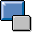Rework in Cost Object ControllingIntegration of Rework for Production Orders

This section explains how rework is integrated in the system and its effects on Cost Object Controlling:

• Individual operations

You manually insert individual operations at the appropriate point in the production order.

• Reference operation set

You enter a reference operation set in the existing order.

The planned costs are then recalculated. The costs are updated on the original order when the operations you inserted are confirmed.

If scrap is confirmed on a rework operation, the Cost Object Controlling component treats that operation as if the scrap had occurred on the operation that caused the rework. The costs of the components and activities are consequently not part of the scrap but go into the usual variance categories.

• Separate production order

You create a separate production order without reference to a material.

In this case the costs for the rework are updated with the confirmation of the operations on the new order. When you settle, these costs are passed on to the original order.

When you calculate the variances, the settled costs of the rework order are shown in the original order under the usual variance categories.

If you settle the rework costs of the separate production order under a settlement cost element to the original production order, you can identify the rework costs through the settlement cost element. In the variance calculation process, the rework costs are reported as a resource-usage variance in this case.

Note that the receiving production order should not be deleted or archived until the rework order has settled to the original production order.

Rework with Trigger Points

Trigger points are useful when rework frequently occurs at certain points in the manufacturing process. Trigger points allow you to integrate reference operation sets or create separate production orders for rework. You achieve this by:

• Changing the status of the operation
• Confirming an operation Here the user decides whether the function (in this case, rework) is triggered.

How Do I Plan Rework?

You can plan rework by defining operations for rework in the routing. Set the Rework indicator in the control key of the operation to differentiate these operations from the other operations. You can manually enter an operation quantity for these operations in the production order.

• When the planned costs are calculated for the production order (preliminary cost estimate for production order), the rework quantity in the operation is taken into account to calculate the variable costs. If the operation quantity is zero, the system only calculates fixed costs.
• In product costing the system uses an operation quantity of zero. Only the fixed costs appear in the product cost estimate.